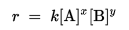# Concept of Collision theory

Collision Theory was introduced in the 1910s by Max Trautz (Trautz, 1916) and William Lewis (Lewis, 1918) trid to account for the magnitudes of rate constants in terms of the frequency of molecular collisions, the collisional energy and the relative orientations of the molecules involved in the collision.

The theory used to predict the rates of chemical reactions, particularly for gases. The collision theory is based on the assumption that for a reaction to occur it is necessary for the reacting species (atoms or molecules) to come together or collide with one another.

### The important parts to collision theory are as follows:

That reacting substances must collide,

That they must collide with enough energy

That they must collide with the correct orientation.

The rate law or rate equation for a chemical reaction is an equation that links the reaction rate with concentrations or pressures of reactants and constant parameters (normally rate coefficients and partial reaction orders). For many reactions the rate is given by a power law such as :where [A] and [B] express the concentration of the species A and B, respectively (usually in moles per liter (molarity, M)). The exponents x and y are the partial reaction ordersand must be determined experimentally; they are often not equal to the stoichiometric coefficients. The constant k is the rate coefficient or rate constant of the reaction.

### Determining Exponents for a Rate Law from Initial Rates (Experimental Data)

If we are given data from two or more experiments at the same temperature with different concentrations of reactants and different rates we can determine the exponents in the differential rate law for the reaction as follows:

1.Write the rate law with the concentrations of all species for which data is given. Write the coefficients as unknowns: n, m, etc. For example, we might have an equation such as the following

rate = k[A]n[B]m

ratios of the experimental data that give different rates

rate1 / rate2 = k[A]n1[B]m1 / k[A]n2[B]m2